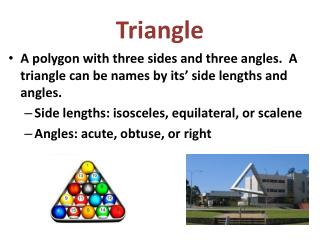DownloadDownload PresentationTriangle

# Triangle

Télécharger la présentation## Triangle

- - - - - - - - - - - - - - - - - - - - - - - - - - - E N D - - - - - - - - - - - - - - - - - - - - - - - - - - -
##### Presentation Transcript

1. Triangle • A polygon with three sides and three angles. A triangle can be names by its’ side lengths and angles. • Side lengths: isosceles, equilateral, or scalene • Angles: acute, obtuse, or right

2. Isosceles Triangle An isosceles triangle has two sides of equal length.

3. Equilateral Triangle A triangle that has all sides of equal length.

4. Scalene Triangle A triangle with no sides of equal length.

5. Acute Triangle An acute triangle has 3 acute angles (all less than 90°).

6. Obtuse Triangle An obtuse triangle has one obtuse angle and two acute angles.

7. Right Triangle A right triangle has one right angle and two acute angles.